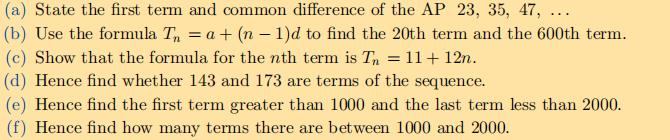### Still have math questions?

Algebra
Question(a) State the first term and common difference of the AP $$23,35,47 , \ldots$$

(b) Use the formula $$T _ { n } = a + ( n - 1 ) d$$ to find the $$20$$ th term and the $$600$$ th term.

(c) Show that the formula for the $$n$$ th term is $$T _ { n } = 11 + 12 n$$ .

(d) Hence find whether $$143$$ and $$173$$ are terms of the sequence. (e) Hence find the first term greater than $$1000$$ and the last term less than $$2000$$ . (f) Hence find how many terms there are between $$1000$$ and $$2000$$ .

$$\left. \begin{array} { l } { \text { 5(a) } a = 23 , d = 12 \quad \text { (b) } T _ { 20 } = 251 , T _ { 600 } = 7211 } \\ { \left. \begin{array} { l l l } { \text { (d) } 143 = T _ { 11 } , 173 \text { is not a term. } } & { \text { (e) } T _ { 83 } = 2007 } \\ { T _ { 165 } = 1991 } & { \text { (f) } 83 \text { (Count both } T _ { 83 } \text { and } T _ { 165 } \text { . } } \end{array} \right. } \end{array} \right.$$